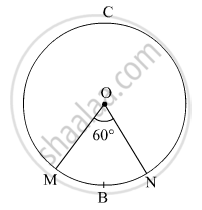Share

# In the Given Figure, the Radius of the Circle is 7 Cm and M ( Arc Mbn) = 60°, Find Area of the Circle. - Geometry

ConceptPerimeter and Area of a Circle

#### Question

In the given figure, the radius of the circle is 7 cm and m ( arc MBN) = 60°,
find  Area of the circle.#### Solution

Radius of the circle, r = 7 cm
m(arc MBN) = ∠MON = θ = 60º
Area of the circle = $\pi r^2 = \frac{22}{7} \times \left( 7 \right)^2$  = 154 cm2

Area of the circle = 154 cm2

Is there an error in this question or solution?

#### APPEARS IN

Balbharati Solution for Balbharati Class 10 Mathematics 2 Geometry (2018 to Current)
Chapter 7: Mensuration
Practice set 7.3 | Q: 6.1 | Page no. 154

#### Video TutorialsVIEW ALL 

Solution In the Given Figure, the Radius of the Circle is 7 Cm and M ( Arc Mbn) = 60°, Find Area of the Circle. Concept: Perimeter and Area of a Circle.
S